# Acta Universitatis Danubius. Œconomica, Vol 12, No 1 (2016)

The Impact of Consuming Petroleum Products on Economic Growth and Regional Convergence in Iran

Teymur Rahmani1, Kolsoom Roshani2, Masoume Emami Kalaee3

Abstract: One of important subjects considered in models of economic growth is convergence hypothesis. It posits that if different regions have identical levels of log-term equilibrium per capita GDP, the poor regions would have higher rate of per capita GDP growth than rich ones. Therefore the more poor regions would converge towards richer regions in terms of economic conditions. However, since determining factors for long term per capita GDP is not the same in all of regions, the conditional convergence is suggested. This hypothesis states that farer regions from long term per capita GDP would have higher rate of per capita GDP. Since determining factors on economic growth can influence on convergence process, the impact of consuming petroleum products as a determining variable on economic growth has been studied. The model of Barro and Sala-I-Martin was applied in order to examine convergence and the impact of consuming petroleum products on convergence and also reduction of regional inequality among Iran's provinces from 2000 through 2011. Results indicated that there is an unconditional convergence among Iran's provinces and also variables of gasoline, diesel, and Mazut (fuel oil) have significant impact on economic growth and lead to negative convergence among Iran's province. Hence, respecting these results it can be concluded that using energy subsides cannot reduce regional inequality.

Keywords: Economic Growth; Beta convergence; consumption of petroleum products

JEL Classification: E29; O40; R11

1. Introduction

Achieving to economic growth and development has been regarded as one of important economic purposes. According to historical point of view, growth hypotheses was initialized by an article by Ramsay in 1928 and continued by people such as Harrod (1939) and Domar (1946) and then Solow-Swan (1956) completed related issues of neoclassic growth, however growth analyses and implications had not been considered until mid-1980s. By advent of 1970s till 1980s, most of advanced economics and subsequent developing countries got reduction of economic growth and especially growth of efficiency. This subject led to respecting macroeconomic growth analyses, which at one side caused considering endogenous growth models and on the other hand it led to deliberate exogenous growth models or neoclassic growth models theoretically and empirically. Unlike neoclassic growth models that consider technical progress to be as exogenous, endogenous models posits technical progresses to be as endogenous and seek for some factors for explaining knowledge production.

Besides these analyses, convergence hypothesis has been extracted from neoclassic growth model. This hypothesis states that different regions are getting converged towards a level of stable balance. Whether this stable balance is common or different, then the concepts of absolute and conditional convergence are shaped. Absolute convergence states that if different regions have identical long term equilibrium per capita GDP, then the poorer regions (with lower actual GDP) would have higher rate of per capita income growth than richer regions (with higher actual GDP). Therefore, poorer regions get closer to the richer regions in terms of economic conditions. But since determining factors of long term per capita GDP (parameters of growth model) are not the same in different regions, conditional convergence would be posited. This hypothesis states that farer regions from long term per capita GDP would have higher rate of per capita GDP growth.

One of important features of developing countries is the presence of dichotomy among different regions. Some of them, which are big cities, are higher level of development and per capita income would be more. Researchers done in Iran indicate difference among different province in terms of economic development and removal of dichotomy among different provinces and balanced development is among objectives of country's developmental plans. The requisite for achieving to this purpose is higher speed of growth in undeveloped and poor regions towards developed and rich ones, which has been regarded as convergence hypothesis in growth models.

According to various economic schools, work force and capital (including professional and non-professional) are considered as most important factors for economic growth at growth functions. In new growth theories, the factor of energy is also introduced, which is recognized under title of energy carrier including petroleum products, electricity, gas, etc. this hypothesis states that consuming energy carriers are effective on economic growth and subsequently on convergence speed. Therefore, in the case of proving this hypothesis, it can be concluded that injecting indirect subsidies of energy by government into poor regions through keeping prices level low and increasing incentive of consumption can influence upon regional economic growth and speed of regional convergence.

Respecting mentioned issues, the main question of present is raised: is there any convergence among provinces of Iran? And what kind of effect do consuming petroleum products have on convergence among Iran's provinces?

This research examines the hypothesis for Iran's provinces from 2000 through 2011 using the model of Barro and Sala-i-Martin. Remaining parts of study are presented as; first a brief review of growth and convergence would be presented, then a review on previous studies would be provided and finally an introduction of data and hypothesis testing and conclusion would be brought.

2. A Review of Growth and Convergence Theories

One of obtained results from economic growth models id economic convergence hypothesis. It states that economics with lower income towards economics with higher income would tend to faster rates of growth.

Convergence hypothesis is the natural result of neoclassic homogeneous of production function degree 1 towards two inputs of work force and capital. Also, after stating convergence hypothesis of Solo neoclassic growth model, this hypothesis is generated into endogenous growth models. Based on convergence literature, if economics get closer to a stable balance level from per capita income, absolute convergence would be appeared. If they get converged to their stable levels, conditional convergence would be established. According theory of neoclassic models, convergence is examined through income level and growth rate.

Here, convergence hypothesis is examined within theoretical frame of Ramsay model. In this model, it is assumed that households have infinite age; hence those levels of consumption and savings rate would be chosen that would maximize their utility. Therefore, rate of saver is no longer stable and it is a function of per capita capital of k. also, it is assumed that households are the same and grow along with constant rate of n growth, 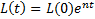. In order to obtain convergence equations in this model, first it is assumed that production function of Cobb-Douglas is presented as follows;

1. 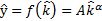

In this relation, y is effective per capita GDP, and k is effective per capita capital. According to this model, household utility function is as follows;

1. 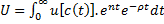

In which is time priority and >0. It is assumed that 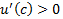and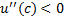.

In close economics, savings are equivalent to investment and since saving is no longer a constant value, hence the equation of effective per capita capital growth can be written as:

1. 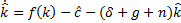

In which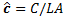, is depreciation rate, g is technology growth rate, n is population growth rate; f (k) is the production function.

Considering instantaneous utility function as (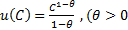 , it is called utility function with constant relative risk aversion or a function with constant elasticity of substitution between periods. By applying first order condition of maximizing utility function of per capita consumption growth rate can be calculated with Euler equation;

1. 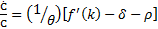

In this equation, the relation between 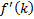 and 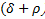 indicates that pattern of household consumption is increasing, decreasing, or fixed. At the state of stable balance, variables of efficient per capita 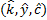have growth rate of zero. Per capita variables of (k, y, c) would grow along with g and level of 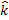 at the state of stable balance can be obtained through the following relation;

1. 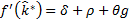

Using log linearization of Eqs:3 and 4, transitional dynamics can be explained near stable balance. In order to determine mean growth rate of y between zero and T, the following relation is applied:

1. 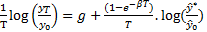

In their researches from 1990 through 1995, Barro- I- Sala applied the following equation for determining  on convergence in European and American states:

1. 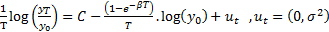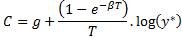In this relation, 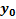 refers to per capita income in 1st year, T is the number of years during entire period, 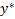 refers to equilibrium per capita income. In Eq.7, intercept of c indicates economy's long term growth rate. In the equation above, positive parameter  indicates balance speed towards stable balance, which is obtained from the following relation:

1. 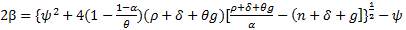

In which; 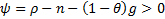

The higher value of  means more interaction between mean growth rate and difference between 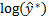 and 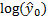. This convergence is running with more speed towards stable balance. In order to make convergence, 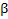 should have positive sign in the Eq.7. it means that reverse relation between initial state and mean growth rate is present during the period. In other words, those regions with lower initial income would have higher growth rate towards regions with higher rate of initial income. However, negative  means divergence of per capita income, hence the gap between poor and rich regions would be higher along with time.

It should be noted that other regional differences are not considered in the mentioned equation. This equation is known as absolute convergence equation, in which coefficient refers to absolute convergence speed among regions. But respecting this point that different region based on many social, economic, political parameters, hence factor c would be different among different regions. Considering other parameters measurable in regression, the equation absolute convergence is converted into conditional convergence as follows:

1. 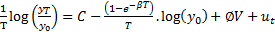

In which V represents vector of other regional variables such as policy (the way of economic management), cultural and social (difference in language, population distribution, et c), regional (geographical situation), economic (income and savings level), and political (degree of political stability and the way of governance) variables. In this equation, conditional convergence and its factor 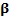 represent speed of conditional convergence among regions.

In order to examine the impact of petroleum products consumption on regional convergence, conditional convergence would be applied, in which consumption of petroleum products are considered as variables at right side of regression.

3. A Review on Experimental Studies

Convergence hypothesis is considered as the most important obtained results from neoclassic growth pattern, introduced in to experimental studies by works of Barro- I-Sala during 1990s. forst studies upon convergence were done for income rates, which examined the relation between per capita growth rate among regions and their per capita initial income. But by appearing some problems on explaining the reasons behind observing different income rates among different regions and countries, some issues related to effective factors on growth were examined.

Bergstrom (1998) examined the process of regional convergence upon 24 regions in Sweden from 1945 through 1990 and confirmed convergence hypothesis of per capita income in these regions. Convergence speed (conditional) was 0.055 indicating removal of 5.5% of gap in per capita income each year.

Zhsng, Litu, and Yao (2001) studied convergence of regional per capita income among China regions applying time series method (considering structural failure or not considering structural failure) for duration 1952-1997. Results showed that each region is converging towards its balance point. In this state, Gini factor was decreasing for each region and Gini factor was increasing among regions. Moreover, by inserting structural failure, they found out a shock in regions' per capita income.

Dunaway, Kaufman, and Steven (2003) studied convergence and the role of government policies. This research was done upon provinces in Canada from 1961 through 2000. Results indicated that convergence hypothesis is confirmed for these provinces. In order to show conditional convergence and the role of government policies, the system of instantaneous equations were applied,

Pedroni &Yao (2006) examined regional inequality among China provinces applying panel data model. Evidences showed that since economic reforms of free trade from 1978, China provinces have had economic growth and divergence has been increasing among these provinces.

Fleisher and Zhao (2010) have studied the impact of human capital, infrastructure capital, foreign direct investment (FDI) on regional inequality and economic growth in China. Results indicated that investment on infrastructures had higher outputs in eastern regions of China and human capital has had a positive effect on production and efficiency growth of production factors among provinces. In whole, investment on human capital would reduce regional inequality in less developed regions and foreign direct investment would have more impact on production prior to 1994 than after that, due to increasing support and accomplishments of private and semi-private business units.

4. Model Estimation

In this part, required data for estimating model would be explained based on experimental models and a brief description upon the way of collecting them would be presented. Next, based on experimental model and present data, the model would be estimated and obtained results would be explained.

4.1. Data Explanation

During present study, sectional data for 28 provinces in Iran would be applied. Data used in this study consists from actual GDP for Iran's provinces and consumption petroleum products. Data on GDP in these provinces was obtained from provincial data for Iran statistical center. Since figures are nominal and in spite of inflation, it is possible to observe misleading data, hence a price index is needed for deflating in each province, for which consumption price index (CPI) was obtained from annual statistics of central bank. On the other hand, since convergence test is conducted using per capita data, related data for provinces' population was collected for statistics center. Then, by dividing actual income in estimated population for each province, the value of actual per capita income is obtained, in Iran; data from GDP from 2000 through 2011 are available. Therefore, convergence is calculated during this period. Since in some models of economic growth consumption of energy carriers are known as one of important growth factors, the variable of consuming energy carriers has been considered in this model, whose data was obtained from international energy study institutions. Respecting data as sectional data, the method NLS is used for estimating model. Some provinces have major petroleum resources. In this regard, a dummy variable is considered in the equation. The figure1 is regarded for these provinces and zero is considered for other provinces. Oil-rich provinces were Ilam, Bushehr, Khuzestan, and Kohkiluyeh and Bouyer Ahmad.

4.2. Experimental Estimation

Since, households and factories of regions in a country incline towards a similar culture and technology and all of regions have a common central government, hence they would have shared institutions and legal system. Therefore, non-conditional convergence or absolute convergence among different regions of a country towards other countries is more likely. First, regardless regional differences, absolute convergence can be estimated among Iran's provinces using Barro and Sala -I-Martin method as follows;

1. 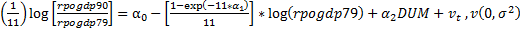

In this relation, 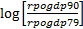 represents actual rate of per capita income growth at the end of period towards actual per capita income from the beginning of period and 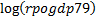 refers to actual per capita income log form the beginning of period and DUM is a dummy variable for oil-rich provinces and 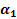 is speed of absolute convergence. Obtained results from estimation are presented in the following table.

Table 1. Results of non-conditional convergence with Barro and Sala -I-Martin method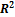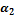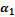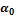equation 33/0 057/0 (09/3) 052/0 (38/2) 203/0 (87/10) Equation.10

Source: Research finding

Figure in the parentheses represent t value for coefficients that by comparing them with present values in the table t, it can be claimed that coefficient rpogdp79 has required significance level. Therefore, first there are some evidences implying absolute convergence among Iran's regions. Factor of convergence speed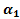 is positive with value of 0.052. Hence, the relation between initial state of provinces and their growth has been negative; hence poorer provinces have more growth than richer provinces. Therefore, according to definition of absolute convergence, these provinces are converging towards a common level of stable balance. Second, convergence speed among provinces equals 0.052. It means that 5.2% from gap between regions is reduced to the balance point. Petroleum dummy variable is positive and significant; indicating that availability of oil in some provinces has a positive effect on growth in these provinces. Obtained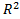 indicates that only 33% of changes in actual per capita growth rate are justified by initial actual income rate. Therefore, other factors are effective in explaining growth, which have not been elaborated here. One of them is energy consumption. In order to answer whether variables of consuming petroleum products (gasoline, diesel, Mazut (fuel oil)) are effective on process of convergence, it is needed to examine individual effect of each on economic growth and speed of convergence. As explained before, economies don’t have equal parameters and it may be different rates of savings or other various parameters. Hence, appeared convergence is called conditional convergence. In order to estimate this kind of convergence, different variables can be inserted at the right side of equation. These represent other factors effective on per capita production growth rate. In this regard, the variable of per capita consumption of petroleum products would be considered individually for examining the impact of each variable on actual per capita convergence and growth. Final equations are as follows and respecting results for each are provided in table.2.

(11) 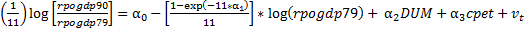

(12) 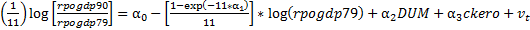

(13) 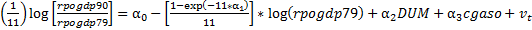

(14) 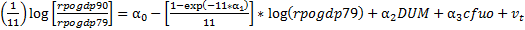

In these relations, Cpet refers to mean of per capita gasoline consumption, ckero is mean of per capita consumption for Kerosene, and cgaso is mean of per capita consumption for gasoil, and cfuo implies mean of per capita consumption for fuel oil. Estimation results of coefficients are presented in the following table.

Table 2. Results of the effect of petroleum products consumption on convergence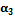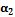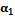Intercept 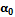 Equation 45/0 22/0 (27/2) 073/0 (94/3) 097/0 (31/2) 16/0 (68/6) Equation11 37/0 11/0- (21/1-) 059/0 (2/3) 065/0 (36/2) 22/0 (30/8) Equation12 46/0 05/0 (45/2) 06/0 (56/3) 055/0 (67/2) 18/0 (34/9) Equation13 39/0 034/0 (56/1) 065/0 (48/3) 061/0 (52/2) 2/0 (022/11) Equation14

Source: Research Finding

Figures in the parentheses imply t value for coefficients that by comparing them with present values in the table t it can be claimed that at error level of 5%,
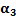 in Eqs.11 and 13 is significant showing effect of consumption variables of gasoline and diesel and at error level of 10%,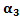in Eq.14 implying effect of fuel oil consumption on growth is significant and positive sign indicates a direct relation of these variables with provinces' income growth. However, the effect of consuming kerosene on convergence (factor in Eq.12) is insignificant. By considering consumption of kerosene in Iran it cab ne observed that domestic and industrial sectors have the lowest and the highest level of final consumption of kerosene, 90.3, 0.5 respectively and since the effect of domestic sector is very trivial in GDP, hence increase of consuming petroleum products has not significant effect on economic growth. Consuming gasoline, diesel, and Mazut (fuel oil) in transportation and industrial sectors is significant, which can lead to increase provinces' income. Since per capita consumption in rich provinces is usually higher than poorer ones, hence it mean that divergence and more gaps can be appeared among provinces and as observed by considering these variables, speed of convergence increases from 0.053 to a higher value. Increase of 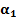in Eqs.11, 13, 14 imply negative effect on convergence between provinces.

Now by analyzing obtained results, in can be concluded that in spite of absolute convergence between regions, variables of consuming petroleum products as one of effective factors on economic growth not only has not reduced income gap, but also it has increased divergence. Hence, it can be claimed that subsides in the energy sector applied by government through reducing regional inequality have cause reduction of convergence with the aim of reducing prices for increasing energy consumption. It is obvious that by paying energy subsides by government; acceleration of convergence would not be effective because richer regions have higher rate of consumption and achieve higher rate of economic growth.

5. Conclusion and Suggestions

In examining the process of convergence between Iran's provinces and by respecting obtained results from estimated model, presence of non-conditional convergence between provinces was confirmed. Also, it was observed that provinces with speed of 0.052 per year are converging towards an equal level of stable balance, by which it would take 13 year to remove half of gap between current level of actual per capita income and stable balance level.

In examining the impact of energy carriers on convergence, variables of consuming different kinds of energy carriers were added to the model. In the case of positive impact of consuming energy on convergence, it can be concluded that energy carriers result in reducing prices, through which increase of energy consumption would be observed that can have a positive significant impact on convergence. Finally, results showed that variables of consuming gasoline, diesel, and fuel oil have a positive significant impact on economic growth and convergence coefficient would be increased. However, since consuming these products in richer regions is higher than poor ones, hence it has a negative effect on convergence and reduces convergence. Therefore, it is obvious paying subsides by government cannot be effective on acceleration of convergence. It is suggested that since consuming petroleum products cause reducing convergence, hence reducing energy subsides may prevent wasting resources and also by substituting energy subsides for required construction costs for each province with the aim of using untouched capacities in the region economic growth and convergence acceleration would be possible.

6. References

Akbari, Nematollah, Moayadfar, Rozita (2003). An examination On Convergence of per capita Income between Iran's Provinces (A spatial econometric approach). Economic Researches Quarterly, No. 13, pp.1-13

Asgari, Hashmat Ullah (2004). Examining the Role of Government Policies in Regional Convergence in the provinces of Iran. Yhesis, guidance by Tymur Rahmani, Tehran University, Faculty of Economics.

Barro, R. J. & Sala-i-Martin, X. (1992). Regional Growth and Migration: A Japan-United States Compariso. Journal of the Japanese and International Economics 6, 312-346.

Barro, R. J. & Sala-i-Martin, X. (1995). Economic Growth. The MIT Press.

Bergstrom, F. (1998). Regional Policy and Convergence of Real per Capita Income among Swedish Counties. SSE/EFI Working Paper Series in Economics and Finance, No. 284.

Cardenas, M. & Ponton, A. (1995). Growth and convergence in Colombia: 1950-1990. Journal of Development Economics, Vol. 47, 5-37.

Energy Balance sheets (2000). Department of Energy.

Farooqi, Pour (2006). Elham, Examining sigma and beta convergence (absolute) between OPEC (Solow and Swan Hypothesis) 2004-1970. Journal of Business Research, 39, S135-156.

Fleisher, B., Li, H., Zhao, M. Q.(2010). Human Capital, Economic growth, and Regional Inequality in China. Journal of Development Economics 92, 215–231.

Kaufman, M., Swagel, PH., Dunaway, S. (2003). Regional Convergence and the Role of Federal Transfers in Canada. IMF working paper.

Mills, L. & Carlino, G.A. (1996). Testing Neoclassical Convergence in Regional Incomes and Earnings. Regional Science and Urban Economics 26, 565-590.

Pedroni, P. & Yao, J.Y. (2006). Regional Income Divergence in China. Journal of Asian Economics 17, 294–315.

Persson, J. (1997). Convergence across the Swedish counties, 1911-1993. European Economic Review 41, 1835- 1852.

Rahmani, T. & Hasanzadeh, E. (2011). Migration, Economic Growth and Regional Convergence in Iran. Journal of Economic Modeling Research, 2 (5) :1- 19

Rahmani, Teymur (2004). Economic growth and regional convergence in Iran. Journal of Economic Research, No. 66, pp. 155-180.

Rahmani, Teymur & Asgari, Hashmat Ullah (2005). Reviewing government policy on regional convergence in the Iranian provinces applying visual deposits. Economic Research Journal, No. 69, pp. 129-154.

Romer, D. (2001). Advanced Macroeconomics. MacGrow Hill.

1Associate Professor, PhD, Faculty of Economics, Tehran University, Iran, Address: Amir Abad, Tehran, Iran, E-mail: trahmani@ut.ac.ir.

2 PhD student, Faculty of Economics, Bu Ali Sina University, Iran, Address: Hamedan, Iran, Corresponding author: kolsoom.roshani@yahoo.com.

3PhD student, Faculty of Economics, Allameh Tabatabai University, Iran, Address: Dehkadeh-ye-Olympic, Tehran, Iran, Tel.: +98 21 4473 7510, E-mail: masoume.emami@yahoo.com.

AUDŒ, Vol. 12, no. 1, pp. 14-24

### Refbacks

• There are currently no refbacks.